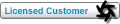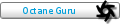## Is it possible to animate vectron values in its script? Tweet

Post, discuss and share handy resources like textures, models and HDRI maps in this forum.
Forum rules
Please do not post any material that is copyrighted or restricted from public use in any way. OTOY NZ LTD and it's forum members are not liable for any copyright infringements on material in this forum. Please contact us if this is the case and we will remove the material in question.

### Is it possible to animate vectron values in its script?

im Wondering if its possible to animate vectron script values by adding a key framable slider or altering its script to say that in x number of frames the number should be x ?

Trying to think a way to animate following numbers on menger sponge boolen.
Mainly the 2.1 and 3.2

vector a = mod( p*s, 2.1 )-1.0;
s *= 3.0;
vector r = abs(1.0 - 3.2*abs(a));

Does anyone know if this is possible?
foliohattuPosts: 1
Joined: Tue Jun 23, 2020 10:21 am

### Re: Is it possible to animate vectron values in its script?

Hi,
you can use the time parameter, please have a look at this example:
Code: Select all
` #include "octane-oslintrin.h"shader OslGeometry(    int Iterations = 10,    float Power = 2.0,    float Bailout = 20,    output _sdf c = _SDFDEF){    vector pos = P;    float power = Power;    float w = fmod(_wipes(), 300.0) / 300.0;    w += time / 300.0;    power = w * 5 + 5.0;   vector z = P;   float dr = 1.0;   float r = 0.0;   for (int i = 0; i < Iterations ; i++) {      r = length(z);      if (r>Bailout) break;            // convert to polar coordinates      float theta = acos(z/r);      float phi = atan2(z,z);      dr =  pow( r, power-1.0)*power*dr + 1.0;            // scale and rotate the point      float zr = pow( r,power);      theta = theta*power;      phi = phi*power;            // convert back to cartesian coordinates      z = zr*vector(sin(theta)*cos(phi), sin(phi)*sin(theta), cos(theta));      z+=pos;   }   c.dist = 0.5*log(r)*r/dr;}`

ciao Beppebepeg4d
Octane GuruPosts: 7314
Joined: Wed Jun 02, 2010 6:02 am
Location: Italy